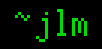## 19-Aug-2010

### Finger Multiplication

Filed under: math — jlm @ 12:39

“Finger multiplication” is an old trick for using the bottom-left (easier to memorize) quadrant of the times table to do multiplication in the top-right quadrant. It’s easy to work out why it works, but unsatisfying, but I found rederiving the technique gave more insight.

How might we do this? To go from one quadrant to the other, we subtract from 10, or generally from the base b: (b-x)(b-y) = b²-bx-by+xy
Well, there’s our xy term, to get it alone we want to subtract off b²-bx-by = b(b-x-y), which we can do by adding -b(b-x-y) = b(x+y-b). The factor of b is trivial to deal with, just use place value, so that leaves us with x+y-b. That’s easy enough to calculate on its own, but we can distribute the -b half to each number as (x-b/2)+(y-b/2). We have the numbers b-x and x-b/2 to do arithmetic on, these sum to b/2, the number of fingers on a hand, so if you hold up x-b/2 (x-5 for us pentadactyl folk) fingers on a hand, then the down fingers number b-x. And that’s how you do finger multiplication, hold up x-5 on one hand, y-5 on the other, put the sum of the up fingers in the tens place and the product of the down fingers in the units place et voilà.

Now that we’ve reverse engineered it for the existing use case, can we come up with something like it for others? How about the case where only one term is > 5 ?
We want to move this from the top-left to the bottom-left of the times table, so x∙(b-y) = bx-xy. So, put the smaller number in the tens place, and subtract off the product of it and the “down fingers” from the large number. 3×7? 7 has 3 down fingers, so 30-9 = 21. 4×8? 8 has 2 down fingers, so 40-8 = 32.